# MAIN

• 1. Introduction

• 2. Specification and Previous Research

•   2.1 AISC Specification

•   2.2 Review of Previous Studies

• 3. New Design Equation

•   3.1 Finite Element Models (FEM)

•   3.2 Non-Linear Regression Analysis

• 4. Experimental Test

•   4.1 Experimental Models

•   4.2 Tensile and Buckling Test

•   4.3 Comparison of Results이틀

• 5. Conclusion

## 1. Introduction

During the past years, significant amount of research has been conducted to assess and develop design guides for the lateral- torsional buckling capacities of stepped beams. Stepped beams are non-prismatic beams designed to have an abrupt increase in cross-section along its critical segments instead of opting to have a prismatic beam of bigger cross-section whenever additional capacity is in need. Such design of steel beams gives the material its aesthetic advantage and economic benefit. For example, in multi-span steel bridges, steps in beams are usually located at end supports where negative bending moments are critical. Stepped beams are categorized as doubly stepped beams (DSB) and singly stepped beams (SSB). DSB refers to continuous beams with increase in cross-section at both ends of the span, while SSB refers to beams with continuous and discontinuous ends where the increase in cross-section is at the continuous end. Stepping of beams is done by increasing the flange dimensions during fabrication for hot-rolled and built-up sections or by adding cover plates at the top and bottom flanges of beams. The dimensions of stepped sections can be determined using the stepped beam parameters: α, β, and γ, corresponding to the relative length, flange width, and flange thickness of large and small cross sections, respectively. The stepped beam parameters are illustrated in Figs. 1 and 2.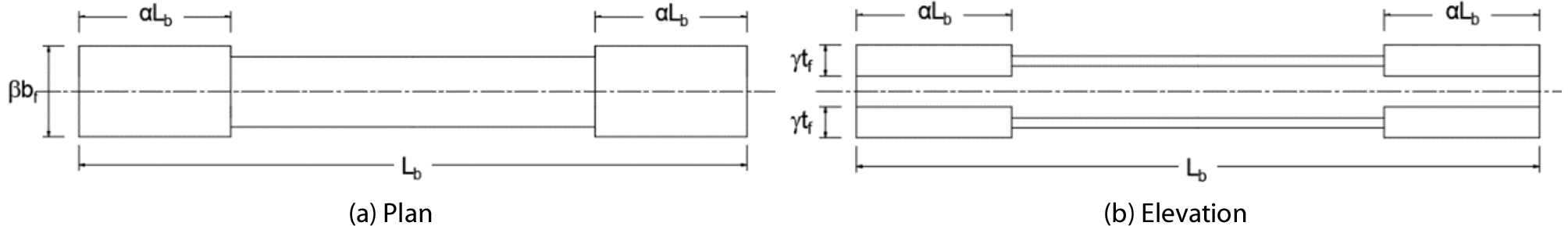##### Fig. 1.

Stepped Beam Configuration for DSB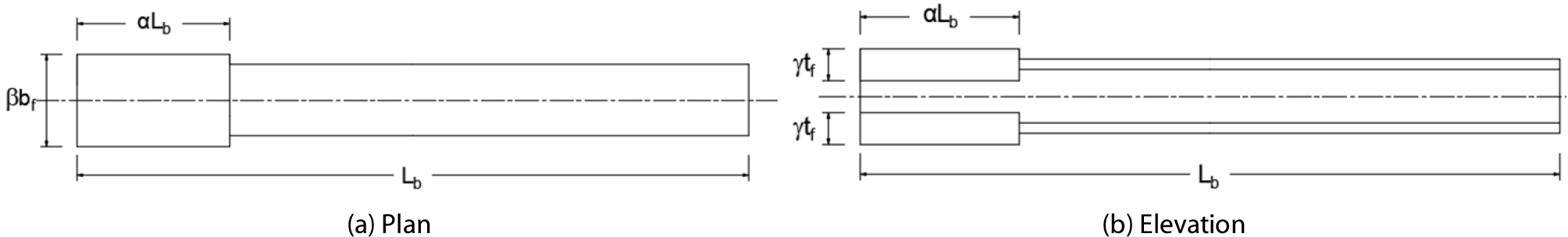##### Fig. 2.

Stepped Beam Configuration for SSB

While non-prismatic beams such as stepped beams are often more efficient than beams of constant cross section, the equations available in the design codes are mostly applicable only for prismatic beams. The most common method in calculating the capacity of a non-prismatic beam is to assume that the beam is prismatic then adapt the larger cross-section throughout the span, which makes the design over-conservative and uneconomical. Because of the lack of provisions, several studies have been conducted to formulate equations for the buckling capacity of non-prismatic beams under several conditions.

In this paper, a new design equation to determine the inelastic lateral torsional buckling capacity of singly symmetric stepped beams with non-compact flanges was proposed. Its reliability was validated by means of actual experimental test, finite element analysis, and design code provision by the American Institute of Steel Construction (AISC).

## 2. Specification and Previous Research

2.1 AISC Specification

The American Institute of Steel Construction (2017) provides design provisions for the nominal strengths, Mn, of prismatic beams. According to the AISC (2017), singly symmetric beams with non-compact flanges are designed according to the least value of the four limit states; compression flange yielding (CFY), lateral-torsional buckling (LTB), compression flange local buckling (CFLB), and tension flange yielding (TFY). This paper focuses mainly on the inelastic lateral-torsional buckling of beams, thus beams used in this study failed according to the limit state of lateral torsional buckling and can be calculated using:

 $$M_n=C_b\left[R_{pc}M_{yc}-(R_{pc}M_{yc}-F_LS_{xc})\left(\frac{L_b-L_p}{L_r-L_p}\right)\right]\leq R_{pc}M_{yc}$$ (1)

where, Rpc = web plastification factor; Myc = yield moment in the compression flange; Sxc = section modulus of the compression flange about the x-axis; Lb = unbraced length of the beam; Lp and Lr are the limiting laterally unbraced lengths for the limit state of yielding and inelastic range, respectively, and Cb = lateral- torsional buckling modification factor for non-uniform moment diagrams, given as:

 $$C_b=\frac{12.5M_{max}}{2.5M_{max}+3M_A+4M_B+3M_C}$$ (2)

where, Mmax = maximum moment along the unbraced segment; and MA, MB, and MC = absolute value of moments at quarter point, centerline, and three-quarter point of the unbraced segment, respectively.

In addition, for singly symmetric beams having a degree of symmetry, ρ, of less than or equal to 0.23, the torsional constant, J, should be taken as zero. The degree of symmetry is defined as the value obtained from Iyc/Iy.

2.2 Review of Previous Studies

Studies have shown that several factors affect the lateral torsional buckling behavior of beams. Some of these were taken from the study of Serna et al. (2006) which focuses on the effects of support conditions on the buckling behavior of beams; and the effects of geometric imperfections on LTB capacities by Galambos (1963), Lindner (1974), and Kabir and Bhowmick (2016). These have been adapted to the study of stepped beams.

Because of the lack of provisions for stepped beams, finite element programs are being used by many researchers for a comprehensive solution in determining the lateral torsional buckling behavior of stepped beams. However, finite element analysis requires much time and effort to perform. This encouraged structural designers to utilize proposed equations as an alternative way to easily calculate the buckling strength of stepped beams. Trahair and Kitipornchai (1971) proposed a tabulated solution for the elastic lateral torsional buckling of stepped beams under a uniform moment. Caddemi et al. (2013) proposed analytical solutions for stepped Timoshenko beams. Stepped beams with different support conditions were also studied. Some of these were stepped beams resting on an elastic foundation by Kulinski and Przybylski (2015) and stepped cantilever beams by Patel and Acharya (2016). Park and Stallings (2003) introduced an equation to calculate the elastic buckling capacity of doubly symmetric stepped beams with compact sections, Eq. (3), using a stepped beam correction factor, Cst, which considers the change in cross-section of beams.

 $$M_{st}=C_bC_{st}M_{cr}$$ (3)
 $$C_{st}=1+6\alpha^2(\beta\gamma^{1.3}-1)\;\mathrm{for}\;\mathrm{DSB}$$ (4)
 $$C_{st}=1+1.5\alpha^{1.6}(\beta\gamma^{1.2}-1)\;\mathrm{for}\;\mathrm{DSB}$$ (5)

where, Mcr = critical LTB strength of stepped and prismatic beam, respectively; α, β, and γ are the stepped beam parameters; and Cb and Cst are the moment gradient factor, from Eq. (2), and stepped beam correction factor, respectively. This has become the basis of study of Kim et al. (2008), Park and Oh (2009) and Gelera and Park (2011) for elastic LTB strength of doubly and singly symmetric compact stepped beams. Park and Park (2013a) and Surla et al. (2013) analyzed doubly symmetric stepped beams with unbraced length falling under inelastic range.

Son and Park (2012) investigated the buckling behavior of doubly symmetric stepped beams having compact web and non-compact flanges. In addition, Nicolas and Park (2016) proved that the ratio of the unbraced length and the overall depth of beam is directly proportional with the inelastic buckling strength of stepped beams.

Previous proposed equations were validated using experimental study by Park and Park (2013b) and Surla and Park (2015) for singly symmetric stepped I-beams with compact flanges subjected to concentrated load and four-point bending, respectively.

## 3. New Design Equation

3.1 Finite Element Models (FEM)

Using a finite element program, ABAQUS (2013), finite element models were developed to investigate the inelastic LTB capacities of stepped beams under uniform moment. Reduced integration linear shell elements (S4R) was used for the modelling of beams because of its capability to provide enough degrees of freedom which gives accurate inelastic buckling deformations.

The beams used were singly symmetric W12x65 and W14x90, which were taken from the AISC specification (2017). These beams have non-compact flange sections and degrees of symmetry varying from 0.3 to 0.9 with an interval of 0.2. The typical beam cross sections are shown in Figs. 3 and 4. The beams are simply supported with uniform moment gradient and with ends fixed against in-plane transverse deflection, out-of-plane deflections and twist rotations but are unrestrained against in-plane rotations and minor axis rotations. Table 1 shows that the selected beams failed according to the limit state of lateral torsional buckling with its corresponding unbraced lengths.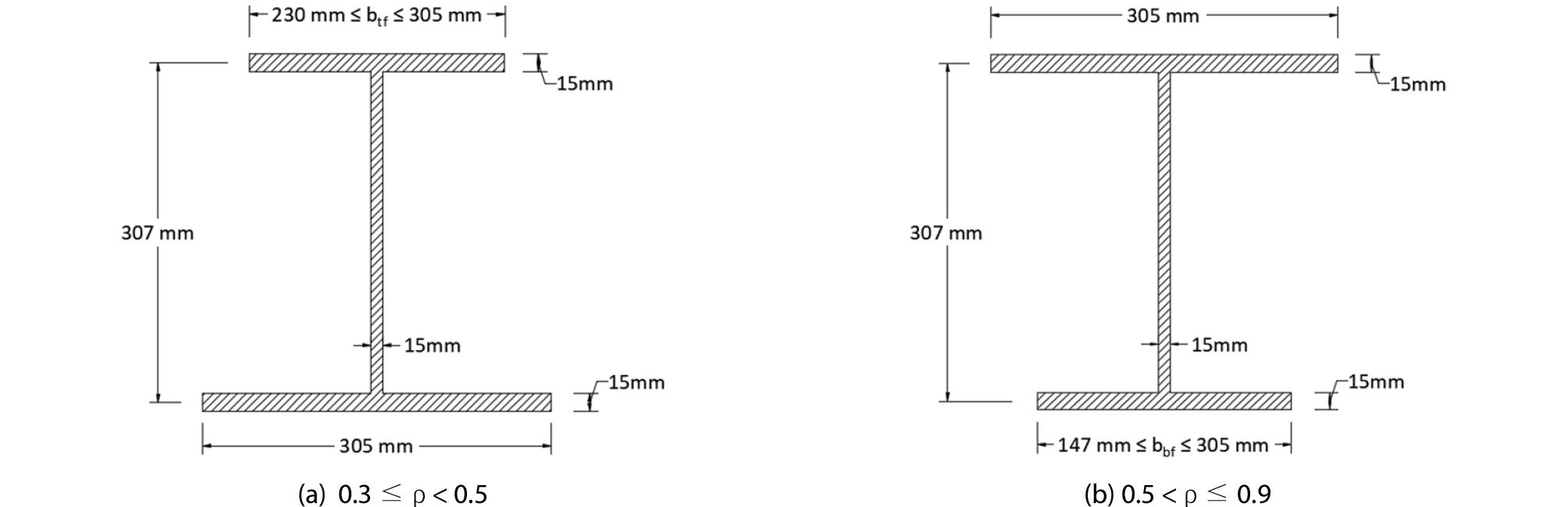##### Fig. 3.

Typical Beam Cross Section of W12x65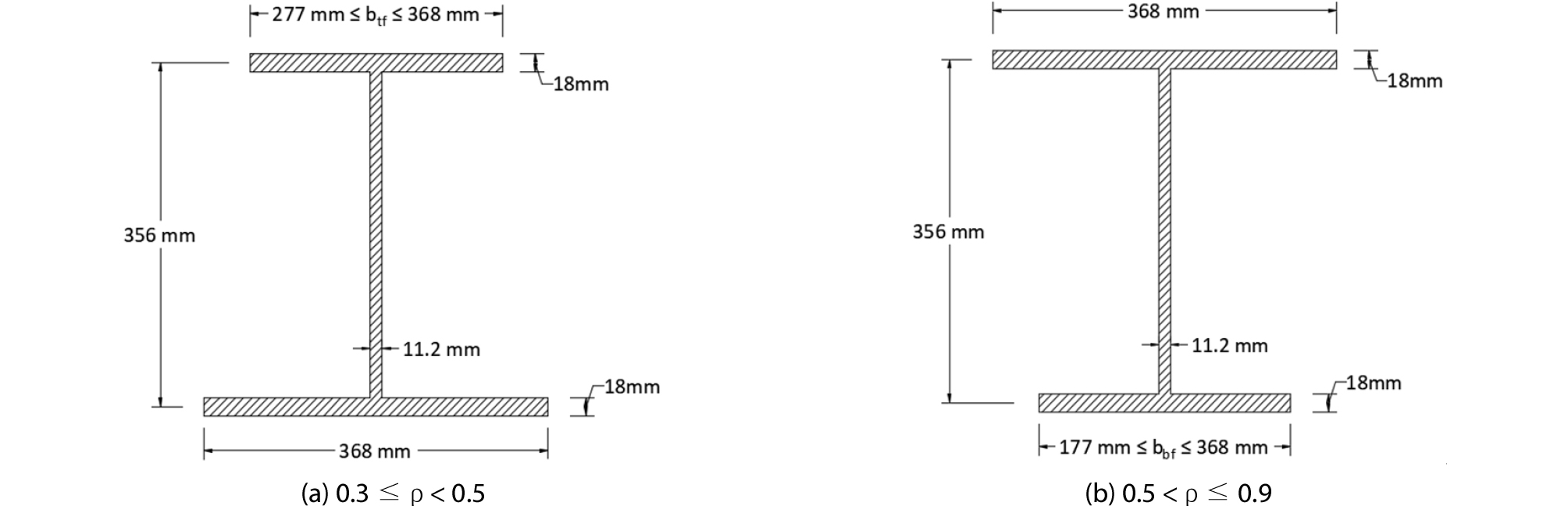##### Fig. 4.

Typical Beam Cross Section of W14x90

Table 1. Strength Parameters of W14x90

 ρ Lb (m) Nominal Strength (kN-m) Mp CFY LTB CFLB TFY 0.3 5000 755.18 628.48 624.97 Not Applicable Not Applicable 7000 565.46 9000 487.95 0.7 5000 755.18 755.18 710.30 743.28 755.18 7000 668.39 9000 626.48 0.9 5000 579.87 579.87 554.93 572.42 579.87 7000 531.45 9000 507.97

Doubly stepped beams and singly stepped beams were analyzed in the study. The stepped beam parameter combinations used were based from the study of Park and Kang (2008), as shown in Table 2. A total of 972 models were performed to develop design equations in calculating the LTB moment resistance of stepped beams. Lastly, beam models were applied with initial imperfection of residual stress from the study of Trahair and Kitipornchai (1971) and geometric imperfection of Lb/1000 taken from the study of Avery and Mahendran (2000). These initial imperfections are shown in Figs. 5(a) and (b).

Table 2. Stepped Beam Parameters

 Deg. of Sym. (ρ) Length (α) Flange width (β) Flange thickness (γ) 0.3 ― 0.9 0.167 1.0 1.2, 1.4, 1.8 1.2 1.0, 1.4, 1.8 1.4 1.0, 1.4, 1.8 0.25 1.0 1.2, 1.4, 1.8 1.2 1.0, 1.4, 1.8 1.4 1.0, 1.4, 1.8 0.333 1.0 1.2, 1.4, 1.8 1.2 1.0, 1.4, 1.8 1.4 1.0, 1.4, 1.8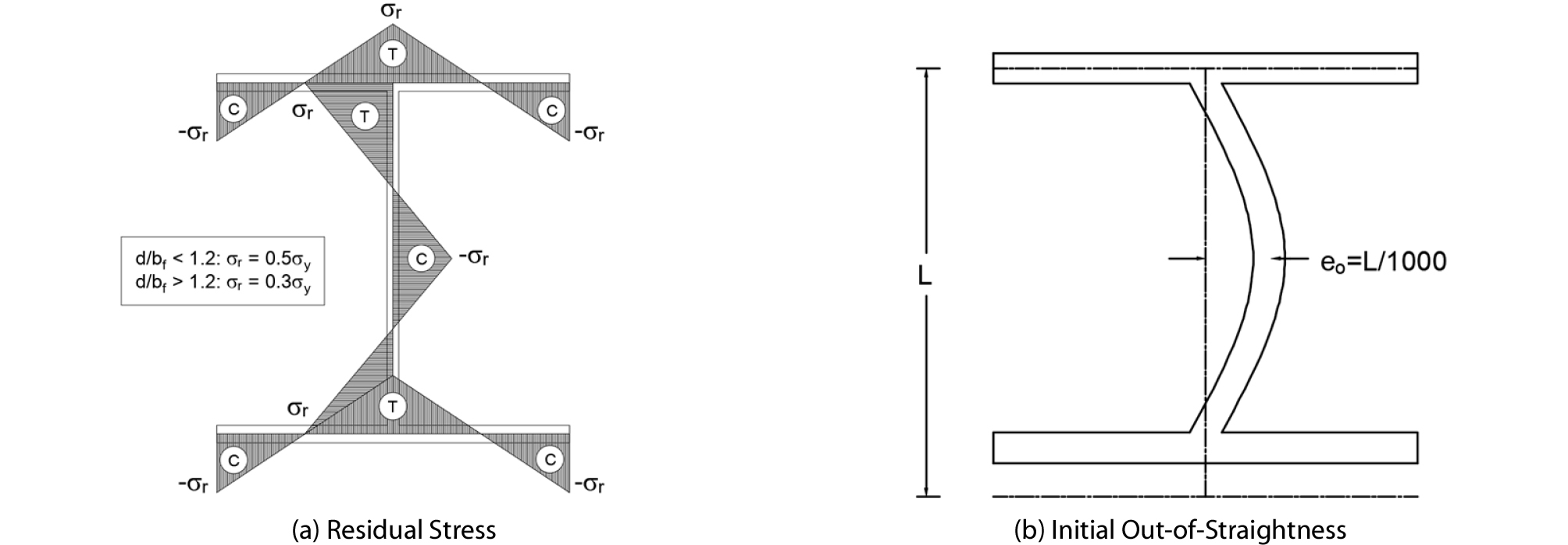##### Fig. 5.

Initial Imperfections

3.2 Non-Linear Regression Analysis

The resulting inelastic buckling strengths from the FEM were transferred to a statistical software, MINITAB 17 (2014), in order to formulate the new design equation. Parameters considered in generating the equation were stepped beam parameters and length-to-height ratio, Lb/h. The proposed inelastic lateral torsional buckling equation for singly symmetric stepped I-beams with non-compact flange sections is:

 $$M_{ist}=C_{ist}M_n$$ (6)
 $$C_{ist}=1+\frac{L_b}{80.9h}(\alpha^{1.7})(\beta\gamma^{2.2}-1)\;\mathrm{for}\;\mathrm{DSB}$$ (7)
 $$C_{ist}=1+\frac{L_b}{190.4h}(\alpha^{3.16})(\beta\gamma^{4.65}-1)\;\mathrm{for}\;\mathrm{DSB}$$ (8)

where, the inelastic stepped beam correction factor, Cist, is defined as the ratio between the inelastic moment capacity of the stepped beam and the prismatic beam with smaller section, which will always be greater than 1.0. This suggests that the inelastic buckling capacity of stepped beams are higher than that of prismatic beams.

Figs. 6(a) and (b) illustrate some of the comparisons between the results of finite element model and Eq. (6) for W12x65 and W14x90. The different shapes represent results from the FEM divided by the torsional buckling of prismatic beams from Eq. (1); and the solid line represents the Cist from Eqs. (7) and (8). The results showed minimal percent differences for both sections with mostly conservative results. The percent differences range from –11 % to 21 % for doubly stepped beams and –12 % to 11 % for singly stepped beams. It is also evident that the highest increase in LTB strength was from the highest Lb/h which is 26.63, illustrating the directly proportional relationship of the length- to-height ratio to the LTB strength discussed in the previous part of this paper.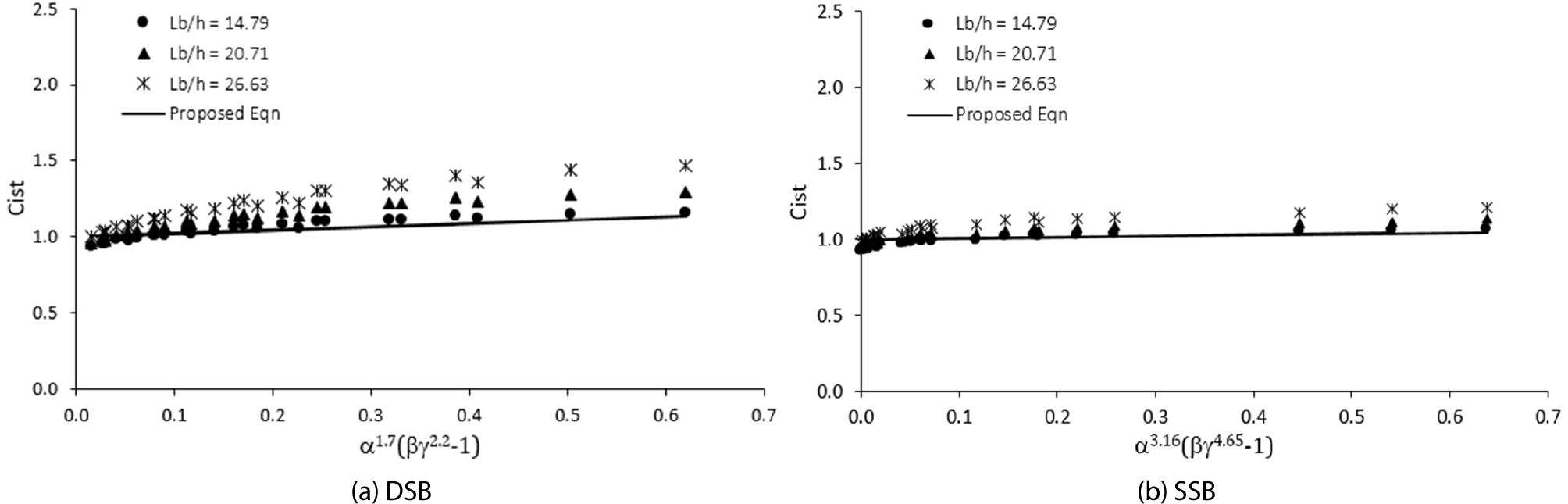##### Fig. 6.

Comparison between Results from FEM and Eq. (6) for W14x90 with ρ = 0.3

To further validate the applicability of the proposed equation on sections used for horizontal structures (such as bridge girders), a W33x118 section with yield strength of 517 MPa and a degree of symmetry of 0.7, was transformed into doubly stepped beams. The buckling capacities of 27 stepped beam cases using FEM and Eq. (6) are shown in Fig. 7. The bar graph represents the results from the finite element analyses and the solid line represents the results using the proposed equation, Eq. (6). It can be seen that the proposed equation yielded conservative results with percent differences ranging from 1.87 % to 7.69 %, proving its applicability on the aforementioned structures.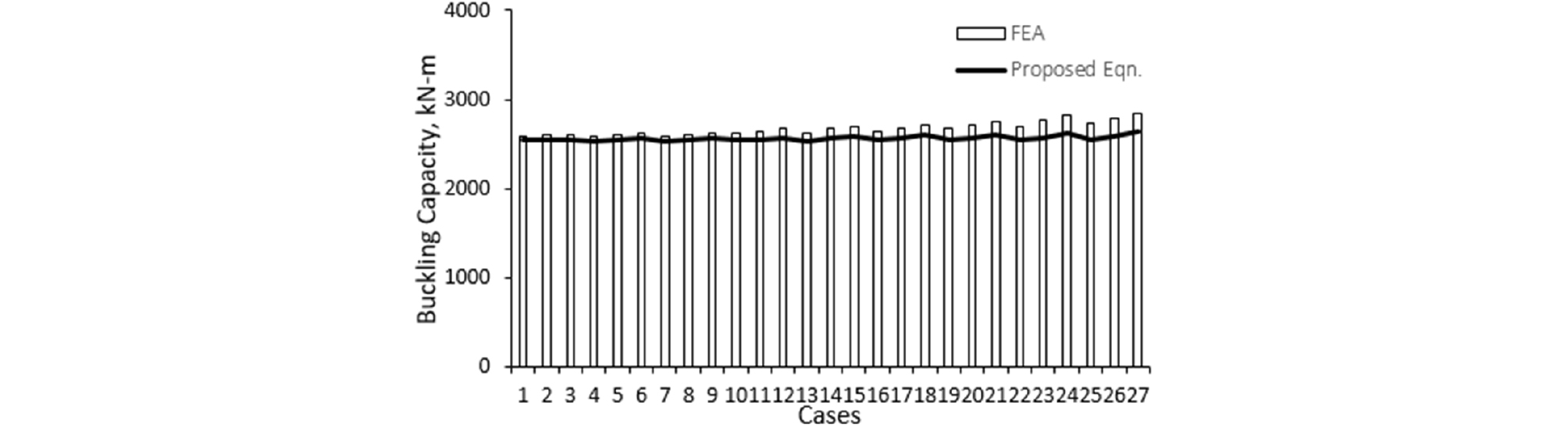##### Fig. 7.

Comparison between Results from FEM and Eq. (6) for W33x118 with Lb/h 4.9

## 4. Experimental Test

4.1 Experimental Models

For the experimental study, four JB300x150 beam sections, taken from the Hyundai Steel manual (2014) for hot-rolled sections, were transformed to singly symmetric stepped beams with a degree of symmetry of 0.7. These singly symmetric beams having non-compact flanges were analyzed to have inelastic behavior since the unbraced lengths of 3 m (SN3), and 4 m (SN4) were under the inelastic range as provided by the AISC (2017). The intermediate values of the stepped beam parameters are given as: α = 0.25, β = 1.0, γ = 1.333 (A) and 1.5 (B). Beam specimens were then labeled as SN3A, SN3B, SN4A, and SN4B. Stepping of beams is done by welding cover plates of the same material on the top and bottom flanges. The cross-section properties of the experimental models are shown Fig. 8.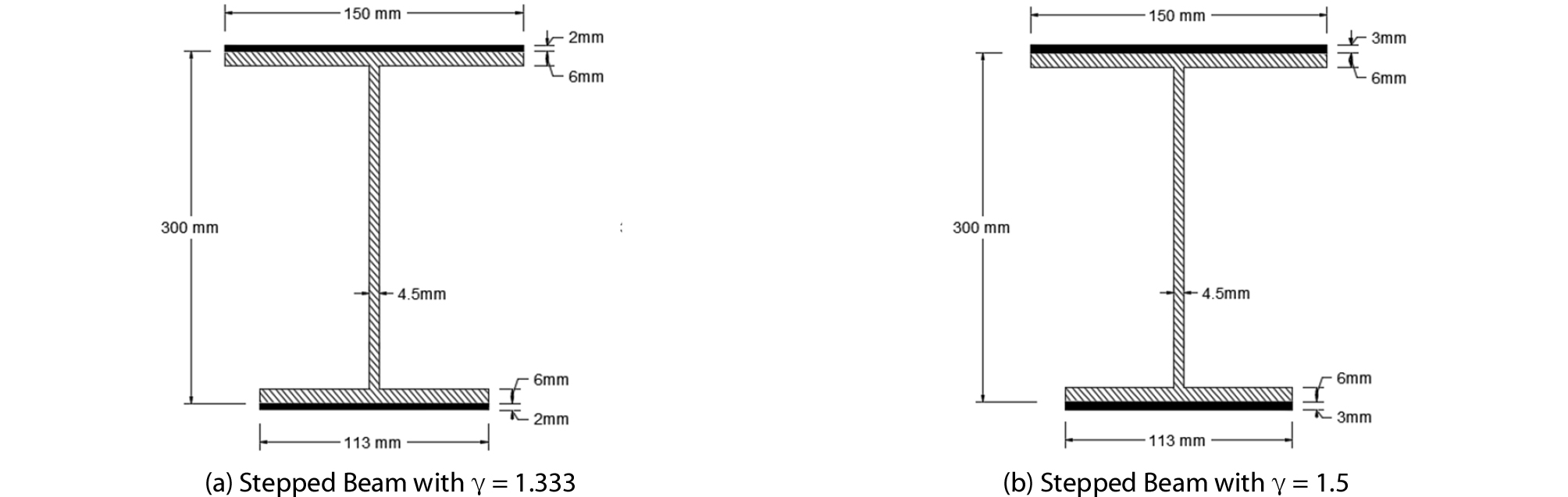##### Fig. 8.

Cross-Section Properties of JB300x150

Fork support condition was used to ensure stability of beams as shown in Fig. 9(a). Roller supports located at 0.1 meters from the end span; and lateral braces located at 0.4 m and 0.5 m from the end span for 3 m and 4 m beam, respectively, are provided. These supports restrained the beam from vertical and out-of- plane displacements near the end supports and allows horizontal displacement when loaded. The loading condition was four-point bending, with the loading frame shown in Fig. 9(b). The loads are 1.0 meter apart from each other, with its center located at middle of the span.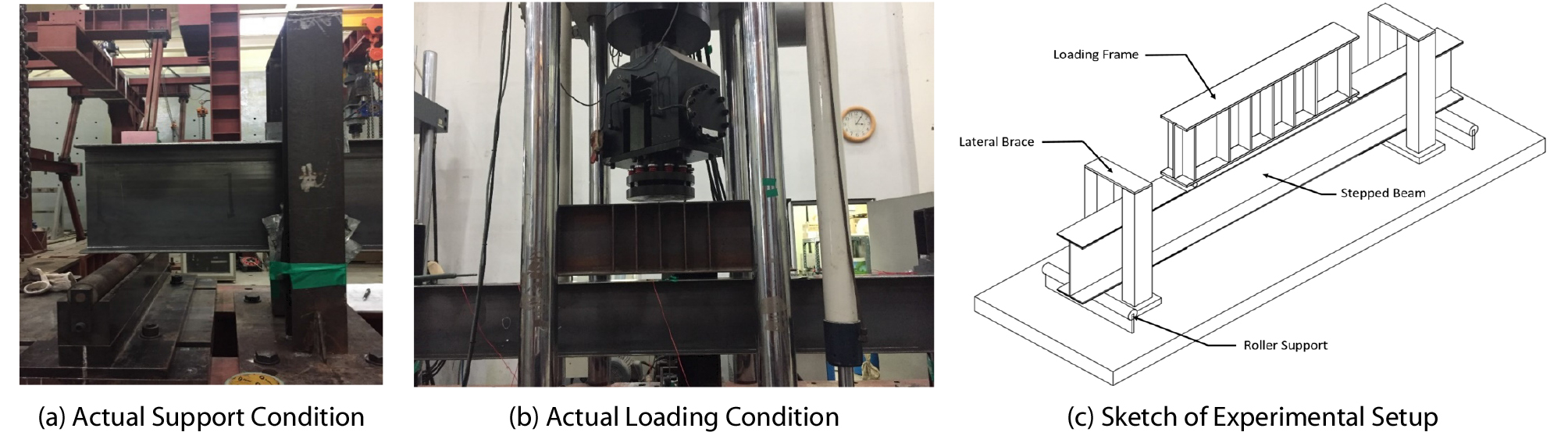##### Fig. 9.

Experimental Setup

4.2 Tensile and Buckling Test

The beam specimens used in the study were fabricated using SS400 steel, with parameters obtained from the Korean Standard KSD3503. These were verified by conducting tensile coupon test following the American Society of Testing Materials (ASTM) E8/E8M. Three coupon specimens were prepared with a gauge length of 100 mm and thickness of 9mm as shown in Fig. 10(a). The specimens were tested using a universal testing machine with a loading rate of 5 mm/min and a loading force of 200 tons. The results of the tensile test are summarized in Table 3.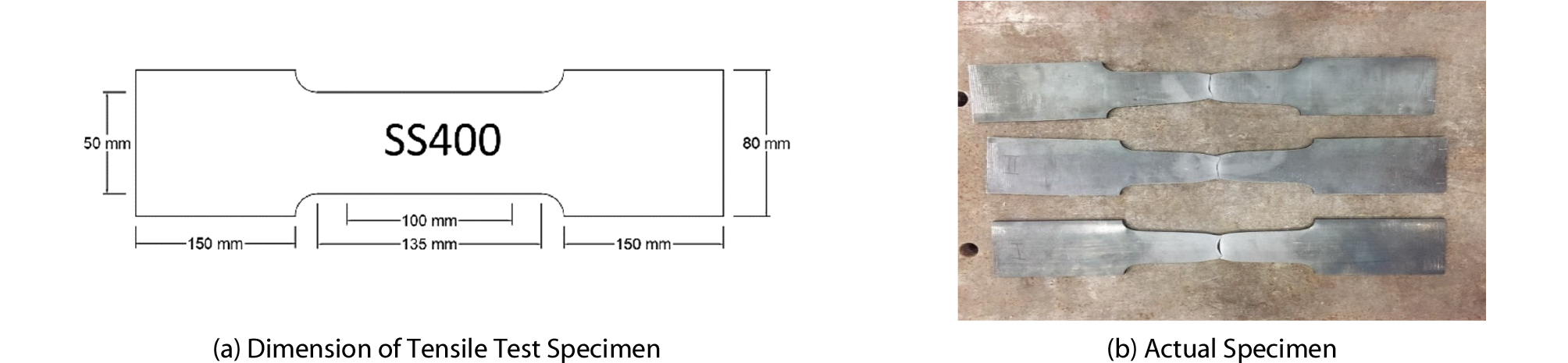##### Fig. 10.

Tensile Test Specimens

Table 3. Summary of Tensile Test Results

 Property SS400 Steel Ave. Test Value KSD3503 Modulus of Elasticity, GPa 196.62 190 ― 210 Yield Stress, MPa 257.68 > 245 Tensile Strength, MPa 414.57 400 ― 510

In preparation for actual lateral bucking test, seven strain gages and two linear variable displacement transducers (LVDTs) were installed to the beam specimens. The strain gages measure the strains experienced by the beam near the stepped flanges and at the center span while the LVDTs measures vertical and horizontal displacement at the center of the beam. Also, strain gages and LVDTs help in monitoring the actual behavior of stepped beams when subjected to two-point load and for further validation of the experimental test. The actual arrangement of the strain gages and LVDTs in the experimental tests are illustrated in Fig. 11.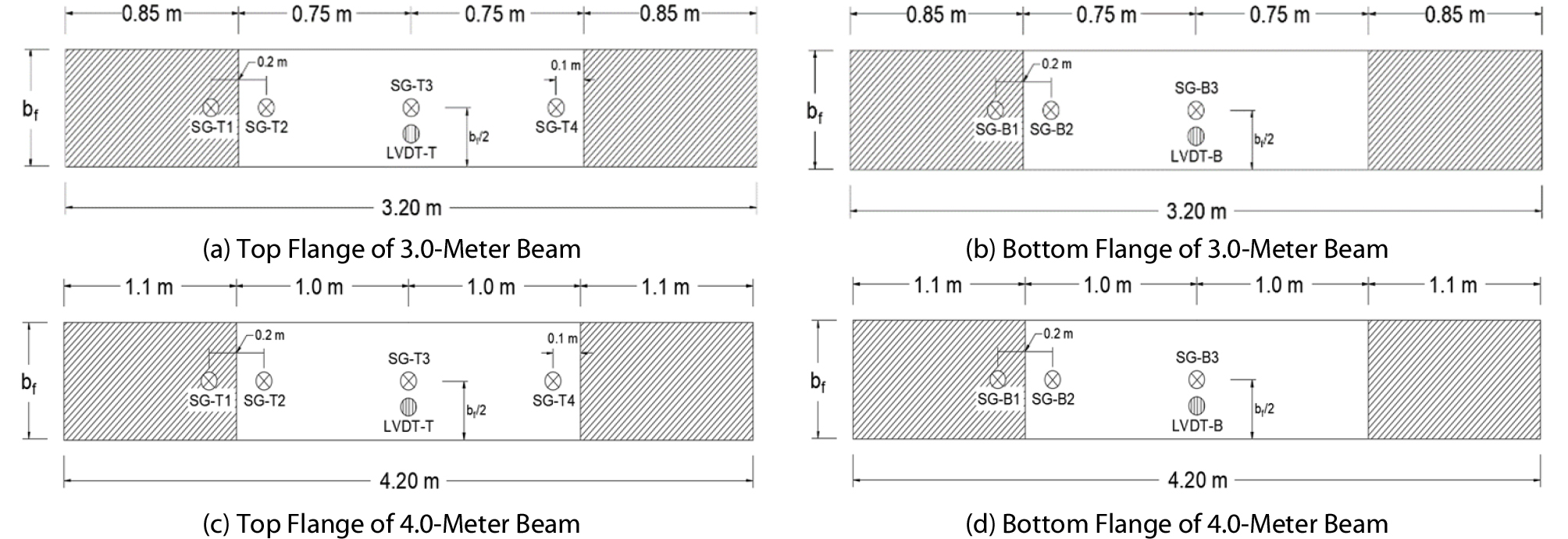##### Fig. 11.

Location of Strain Gages and LVDTs

Lastly, finite element analyses were first conducted to have an initial value of the buckling capacity of beams. These beam models are consistent with the parameters and boundary conditions that will be used in the experimental test. A comparison of the experimental model and FEM is shown in Fig. 12.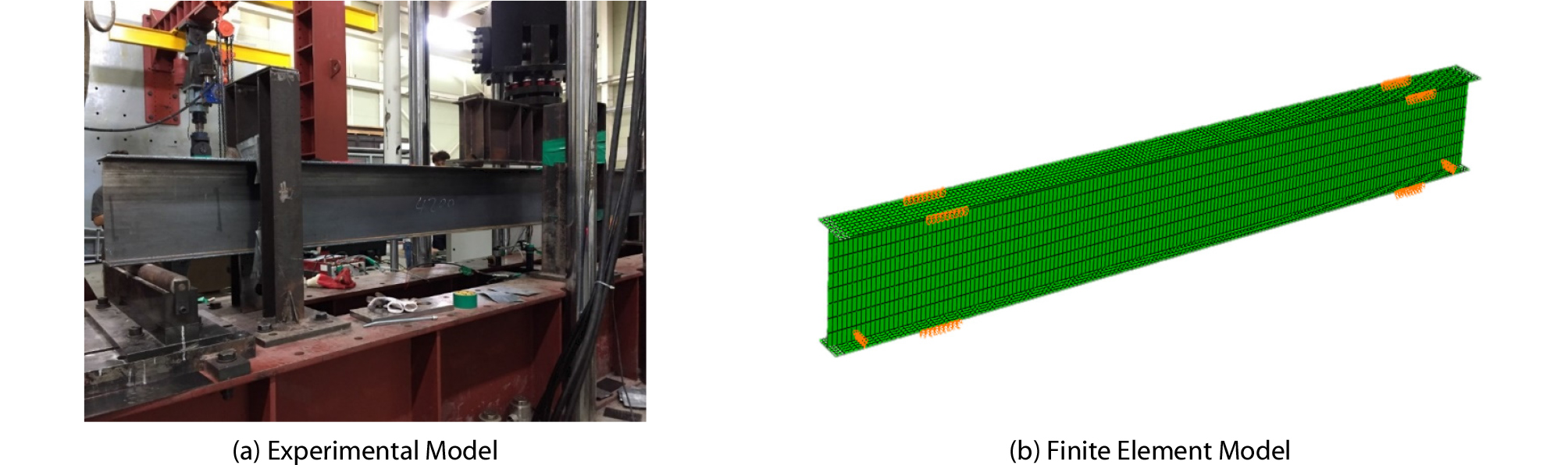##### Fig. 12.

Comparison of the Support Conditions Between Experimental and Finite Element Models

4.3 Comparison of Results

Discussed below are comparisons of the inelastic buckling capacity of beams obtained from the actual buckling test, finite element analyses, equation from AISC, and the proposed equation. For the AISC, the beams are assumed to have the modified cross-sections shown in Figs. 7(a) and (b) throughout the span.

4.3.1 Proposed Equation and FEM

As discussed in the previous part of this paper, finite element analyses were conducted for comprehensive investigation on the buckling capacities of stepped beams. Table 4 shows the comparison between the buckling capacities of the beam specimens generated from FEM and calculated using the proposed equation, Eq. (6). The comparison showed that the proposed equation had a minimal percent difference and with a lower value than that of the FEM, which corresponds to a more conservative estimate. The maximum differences were 1.63 % and 7.83 % for 3 m and 4 m span, respectively. Moreover, an increase in strength is observed as the stepped beam parameter, γ, and the length-to-height ratio increases. This demonstrates the effect of stepping of beams in its LTB capacity.

Table 4. Comparison of Results between the AISC and Proposed Equation

 Length (m) γ Buckling Strength (kN-m) Difference % FEM Proposed Eqn 3 1.0 75.90 - - 1.333 76.20 74.95 1.63 1.5 76.35 75.48 1.14 4 1.0 71.85 - - 1.333 74.25 69.18 6.83 1.5 75.75 69.81 7.83

4.3.2 Experimental Test and FEM

Table 5 shows the comparison between the buckling capacities from the finite element models and the results from the actual buckling test. The maximum difference between the yielded buckling capacities was only about 4.57 %. These show that the values acquired from the buckling test were relatively similar to the experimental test. Fig. 13 shows the buckling mode of the experimental and finite element models.

Table 5. Comparison of Results between the FEM and Test

 Beam Specimen Buckling Strength (kN-m) Difference % FEM Test SN3A 76.2 72.72 4.57 SN3B 76.35 75.60 0.98 SN4A 74.25 72.09 2.91 SN4B 75.75 73.15 3.43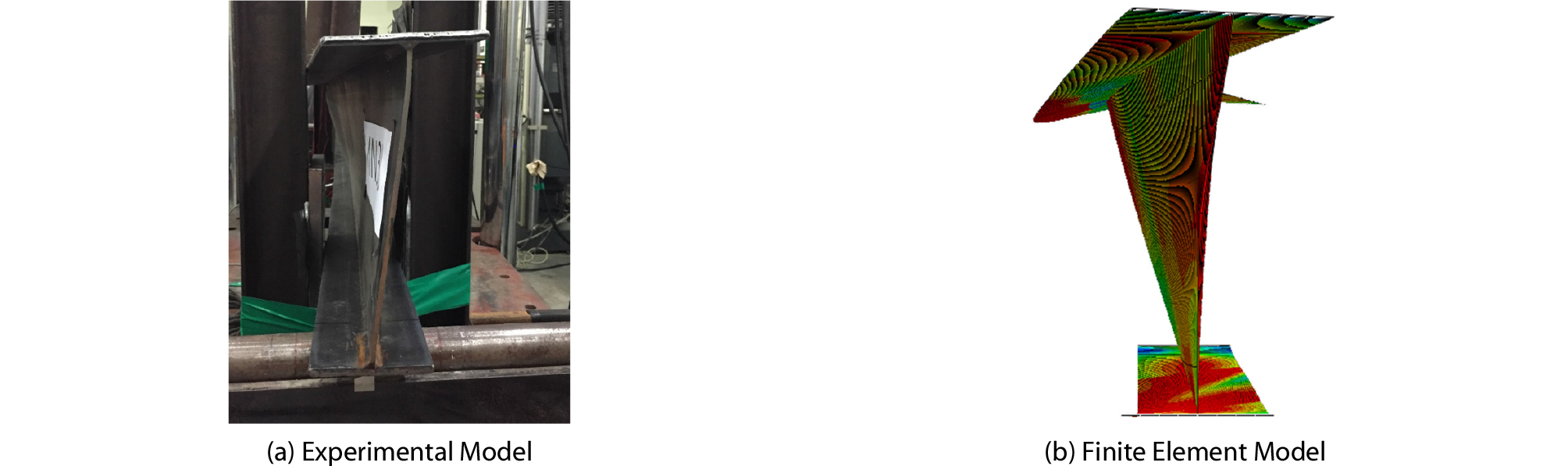##### Fig. 13.

Buckling Modes of Experimental and Finite Element Models

Moreover, the comparisons between the two results are clearly shown in Figs. 14 and 15. In Figs. 14(a)-(d), the load capacities of the beam specimens are graphed with respect to its vertical and horizontal displacements. It can be observed that the beam displaces as the load applied increases and subsequently reached its maximum capacity. Beyond its maximum point, the displacement will continue to increase even without increase in load. Both FEM and experimental test produced a load capacity of 150 kN for 3 m-span beams, and 100 kN for 4m-span beams. This also shows that the beams having longer unbraced lengths have lower capacity than those with shorter unbraced lengths. In addition, Figs. 15(a) and (b) shows some of the strains of the beam specimens graphed against the load capacity. It is observed that the strain values at the experimental test are lower than that of the FEM, which may be caused by external factors while conducting the experiment. Nonetheless, the actual beam specimens were properly simulated using the finite element models.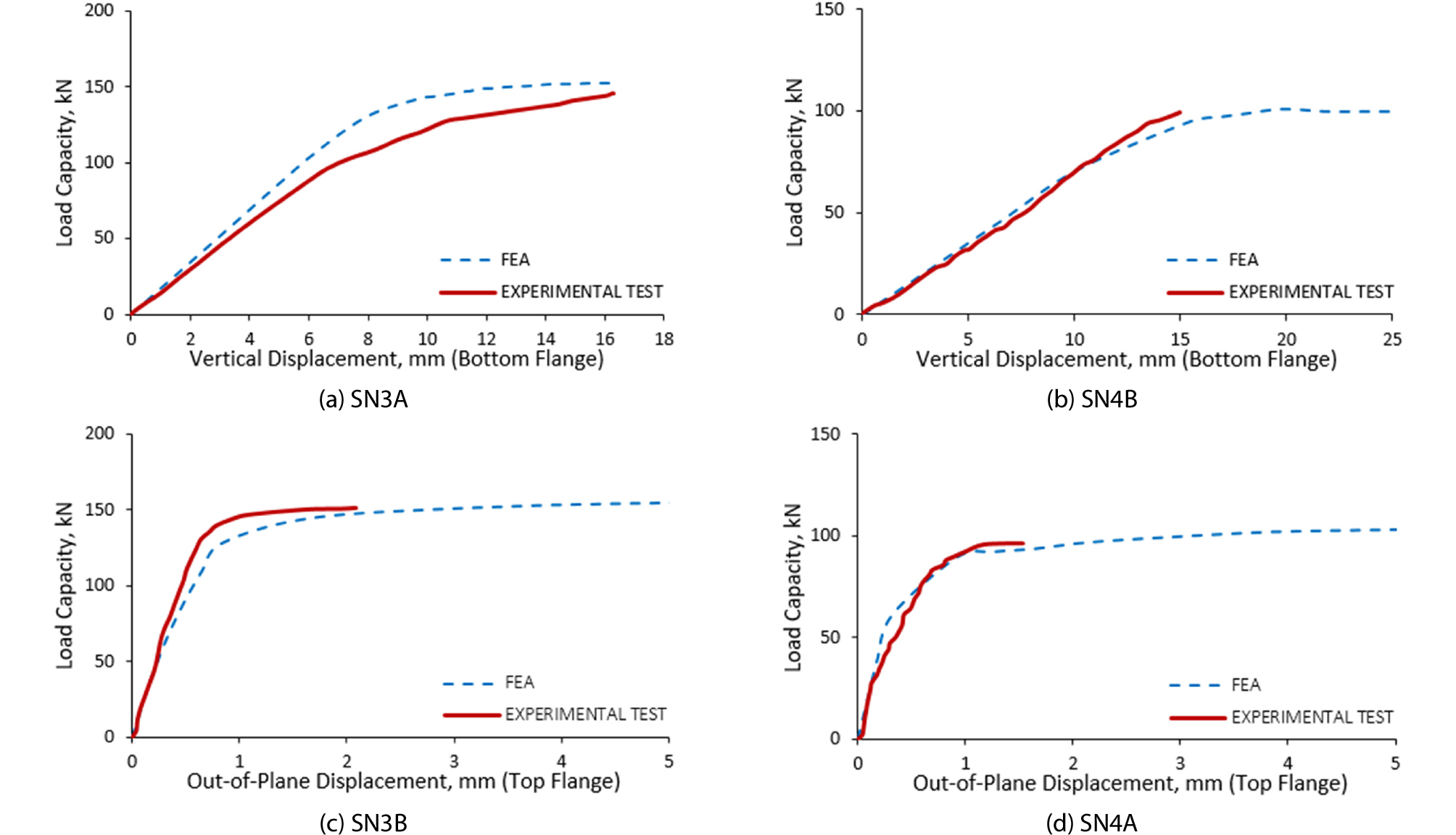##### Fig. 14.

Load-Displacement Curve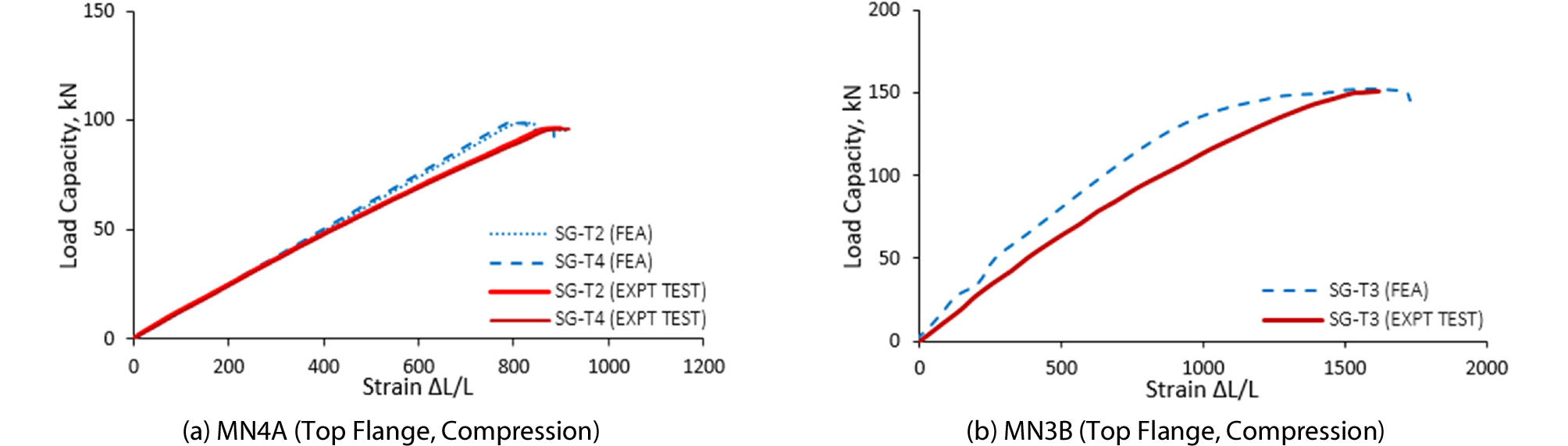##### Fig. 15.

Load-Strain Curve

4.3.3 Experimental Test, AISC and Proposed Equation

The inelastic buckling strength of stepped beams using AISC and the proposed equation were validated for reliability using the results of the actual buckling test. Fig. 16 shows the comparison of buckling capacities gathered from actual buckling test, AISC, and proposed equation. The line represents the results of actual test, while the bar graphs represent the calculated capacities from AISC and the proposed equation. It can be seen that the AISC produced much higher capacity, since it assumes that the beam is prismatic with increased cross-section throughout the span. The percent differences range from 21.32 % to 35.70 % with respect to the actual test results. On the other hand, the proposed equation, which captures the change in cross-section of the beam, produced smaller percent differences ranging from 0.16 % to 4.56 %. It can also be observed that at 4m span, the calculated capacity using the proposed equation is lower than that of the actual test. This demonstrates that the proposed equation gave a conservative estimate of the buckling capacity of stepped beams with non-prismatic flange section.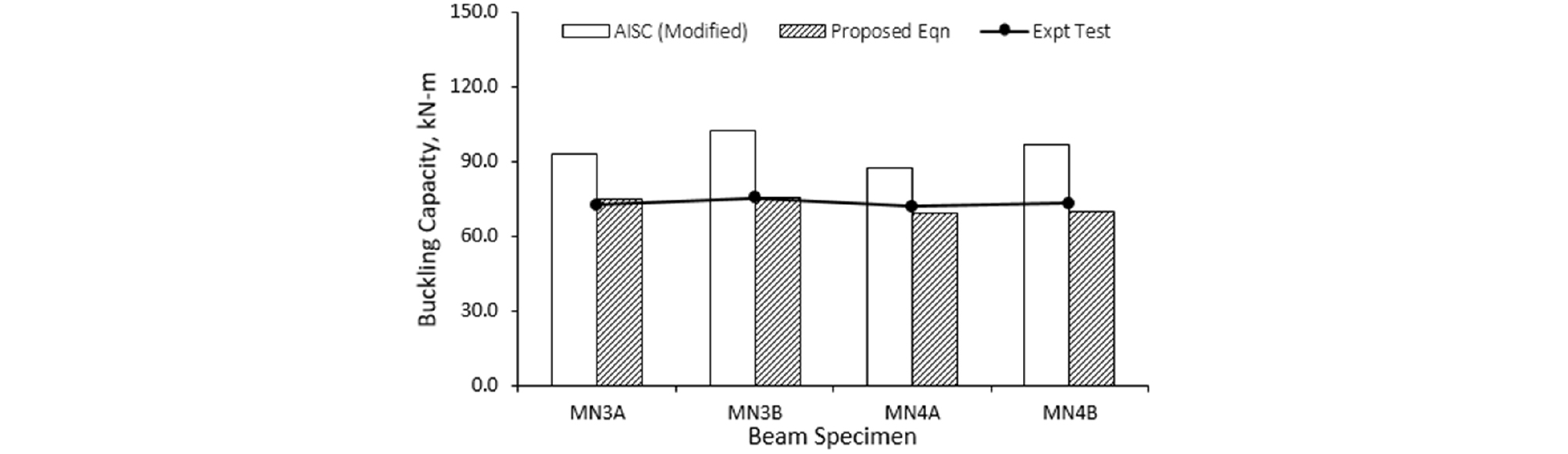##### Fig. 16.

Comparison of Test, AISC, and Proposed Eqn

## 5. Conclusion

The inelastic lateral torsional buckling of singly symmetric stepped I-beams with non-compact flange sections was studied by means of numerical and experimental analyses. The main aim of this study was to generate a proposed equation for calculating the buckling capacity of stepped I-beams using finite element and statistical method. The proposed equation was validated by means of an actual experimental test and finite element models. The proposed equation gave minimal percent difference with conservative estimate when compared with the results of finite element models and experimental models. This proves the applicability and safety of using the proposed equation in calculating the buckling capacities of stepped beams with non-compact flanges. In addition, the use of the proposed equation is much easier and simpler method than using time-consuming and complicated finite element programs. Furthermore, the proposed equation gives a more economical design as compared to the method provided by AISC which tends to be over-conservative when used to non-prismatic beams such as stepped beams.

## Acknowledgements

This research is supported by the Ministry of Land, Transportation and Maritime Affairs (17CTAPC132629-01) of the Korean Government. The funding, cooperation and assistance of various people from these organizations are gratefully acknowledged.

## Acknowledgements

본 논문은 2018 CONVENTION 논문을 수정·보완하여 작성되었습니다.

## References

1

ABAQUS (2013). Standard User's Manual, Version 6.7, Hibbit, Karlsson and Sorensen, Inc.

2

American Institute of Steel Construction (2017). Steel Construction Manual, 15th Edition, AISC, USA.

3

ASTM E8 / E8M (2013). Standard Test Methods for Tension Testing of Metallic Materials, ASTM International, West Conshohocken, PA, www.astm.org.

4

Avery, P. and Mahendran, M. (2000). "Distributed plasticity analysis of steel frames structures comprising non-compact sections." Engineering Structures, Vol. 22, pp. 901-919.

10.1016/S0141-0296(99)00038-3
5

Caddemi, S., Calio, I. and Cannizzaro, F. (2013). "Closed-form solutions for stepped Timoshenko beams with internal singularities and along-axis external supports." Archive of Applied Mechanics, Vol. 83, No. 4, pp. 559-577, DOI: 10.1007/s00419-012-0704-7.

10.1007/s00419-012-0704-7
6

Galambos, T. V. (1963). "Inelastic lateral buckling of beams." Journal of Structural Division, ASCE, Vol. 89, No. 5, pp. 217-244.

7

Gelera, K. and Park, J. S. (2011). "Elastic lateral torsional buckling strength of monosymmetric stepped I-beams." KSCE Journal of Civil Engineering, KSCE, Vol. 16, No. 5, pp. 785-793, DOI: 10.1007/ s12205-012-1255-8.

8

Hyundai Steel (2014). Online Products Guide. Seoul, Korea.

9

Kabir, M. I. and Bhowmick, A. K. (2016). "Effect of geometric imperfection on lateral torsional buckling capacity of I-beams." 5thInternational Structural Specialty Conference, CSCE, London, England.

10

Kim, J. M., Kim, S. J., Park, J. S. and Kang, Y. J. (2008). "A study on inelastic lateral-torsional buckling of stepped I-beams subjected to pure bending." Journal of Korean Society of Steel Construction, Vol. 20, No. 2, pp. 237-246 (in Korean).

11

KS D3503 (2011). Rolled steels for general structures, Korean Standards Association, South Korea.

12

Kulinski, K. and Przybylski, J. (2015). "Buckling of stepped beams resting on an elastic foundation." Journal of Applied Mathematics and Computational Mechanics, Vol. 14, No. 4, pp. 115-126, DOI: 10.17512/jamcm.2015.4.11.

10.17512/jamcm.2015.4.11
13

Lindner, J. (1974). "Influence of residual stress on load carrying- capacity of I-beams." Stahlbau, Vol. 43, pp. 39-45.

14

Nicolas, L. A. and Park, J. S. (2016). "Inelastic buckling capacity of beams considering load height effect, stepped flanges, and compact or noncompact sections." International Journal of Steel Structures, Vol. 16, No. 4, pp. 1135-1148, DOI: 10.1007/s13296-016-0046-3.

10.1007/s13296-016-0046-3
15

Park, J. S. and Kang, Y. J. (2004). "Flexural-torsional buckling of stepped beams subjected to pure bending." KSCE Journal of Civil Engineering, KSCE, Vol. 8, No. 1, pp. 75-82, DOI: 10.1007/ BF02829083.

16

Park, J. S. and Oh, J. J. (2009). "Lateral-torsional buckling strength of monosymmetric doubly stepped I-beam subjected to pure bending." Journal of the Korea Academia-Industrial Cooperation Society, Vol. 10, No. 5, pp. 1020-1025, DOI: 10.5762/KAIS. 2009.10.5.1020.

17

Park, J. S. and Stallings, J. M. (2003). "Lateral torsional buckling of stepped beams." Journal of Civil Engineering, ASCE, Vol. 129, No. 11, pp. 1457-65, DOI: 10.1061/(ASCE)0733-9445(2003) 129:11(1457).

18

Park, Y. S. and Park, J. S. (2013a). "A numerical study on inelastic lateral torsional buckling strength of doubly stepped and singly symmetric I-beam section subjected to uniform moment." Journal of the Korea Academia-Industrial Cooperation Society, Vol. 14, No. 7, pp. 3495-3501, DOI: 10.5762/KAIS.2013.14.7.3495 (in Korean).

10.5762/KAIS.2013.14.7.3495
19

Park, Y. S. and Park, J. S. (2013b). "An experimental study on inelastic lateral-torsional buckling strength of monosymmetric stepped I-beam subjected to a concentrated load." International Journal of Advancements in Computing Technology, Vol. 5, No. 11, pp. 211-216.

10.4156/ijact.vol5.issue11.22
20

Patel, C. M. and Acharya, G. D. (2016). "Analytical determination of deflection of stepped cantilever rectangular beam under uniform load." Journal of Experimental & Applied Mechanics, Vol. 7, No. 2, pp. 1-8.

21

Serna, M. A., Lopez, A., Puente, I. and Yong, D. J. (2006). "Equivalent uniform moment factors for lateral-torsional buckling of steel members." Journal of Constructional Steel Research, Elsevier, Vol. 62, pp. 566-580, DOI: 10.1016/j.jcsr.2005.09.001.

10.1016/j.jcsr.2005.09.001
22

Son, J. M. and Park, J. S. (2012). "Inelastic lateral-torsional buckling strengths of stepped H-beams with noncompact flanges subjected to pure bending." Korean Society of Hazard Mitigation, Vol. 12, No. 1, pp. 53-60, DOI: 10.9798/KOSHAM.2012.12.1.053 (in Korean).

10.9798/KOSHAM.2012.12.1.053
23

Surla, A. and Park, J. S. (2015). "Experimental and numerical investigation on LTB strengths of monosymmetric compact I-Beams with thickness-stepped-flanges." International Journal of Steel Structures, Vol. 15, No. 4, pp. 933-944, DOI: 10.1007/ s13296-015-1214-6.

24

Surla, A., Gelera, K. and Park, J. S. (2013). "A study on the inelastic lateral torsional buckling of doubly and singly stepped I-beam with singly symmetric section subjected to design loading." International Journal of Digital Content Technology and its Applications, Vol. 7, No. 11, pp. 189-193.

25

Trahair, N. S. and Kitipornchai, S. (1971). "Elastic lateral buckling of stepped I-beams." Journal of Structural Engineering, ASCE, Vol. 97, No. 10, pp. 2535-48.